Technical Report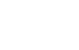Order ratio analysis and tracking analysis
(page1)

Order ratio analysis and tracking analysis

Rotating machines such as engines or compressors etc. are rotating with wide range of rotation speed from low to high speed. For those rotating machines, resonance between rotation speed and natural frequency of each component (rotation shaft, gear, bracket etc.) of rotating machine may be causing serious problem. As an example of torsional vibration including large generator, resonance may generate excitation energy lager than allowable stress, and therefore it causes serious accident to destruction. Rotation-tracking analysis helps greatly to observe the cause such as which component or order of rotation speed makes noise and vibration when a rotating machine makes vibration with certain rotation speed.
As a method for finding these things, "rotational order ratio analysis" and "rotation-tracking analysis" are often used. For rotational order ratio analysis, it defines the phenomenon that occurs once per rotation (one cycle) to be rotation primary order component, n times of the rotation to be n-th order component, and the X-axis shows the order, and Y-axis represents the sound and vibration magnitude.
Rotation tracking analysis performs analysis how the sound and vibration magnitude of the order component that is focused on changes as rotation increases or decreases.
Note: In this case, the order and the order ratio have the same meaning.
The following figure 1 is a conceptual diagram showing the relationship of "frequency analysis", "rotational order ratio analysis" (hereinafter referred to as “order ratio analysis”) and "rotation-tracking analysis" (hereinafter referred to as “tracking analysis) which are measured every 50 r/min increase in rotation speed and displayed in 3D.

• Frequency analysis in 3D: X axis shows frequency (Hz). Components of the same order appear in upper right direction as the rotation increases.
• Order ratio analysis in 3D: X-axis shows order. Components of the same order appear in the vertical direction.

The main difference between Frequency analysis and Order ratio analysis is how it appears on the figure as above when rotation speed increases.

• Tracking analysis: Components of the same order are extracted from each data, and represent the rotation speed on the X-axis, and the order components on the Y-axis. You can see how the order component that is focused on changes as the rotational speed increases. In other words, the data shows that the vibration of the order component of interest gradually increases as the rotational speed approaches the resonance frequency of the component, and becomes maximum when it matches the resonance point, then it decreases gradually after the rotation speed passes the resonance point. From these data, you can easily check the resonance status of components. Tracking analysis mainly includes “constant ratio tracking” and “constant width tracking”.

Firstly, we explain relationship between order ratio analysis and frequency analysis.

 ■ Rotation-tracking analysis conceptual diagram The figure below shows frequency analysis and rotation order ratio analysis are performed and displayed in 3D when rotation speed is made to increase from 850 r/min every 50 r/min and also shows the rotation tracking analysis is performed extracting the 1st order component from 3D data.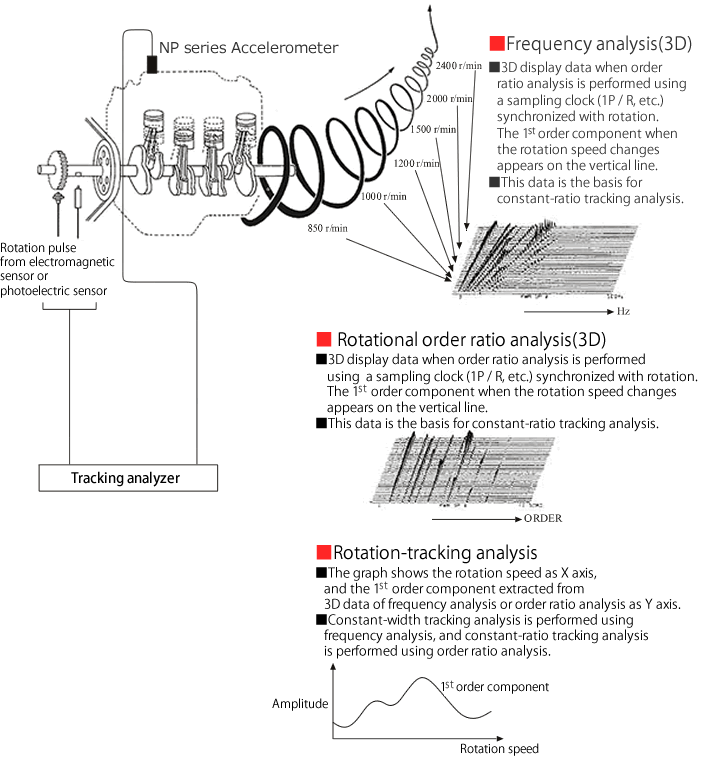Fig. 1

1.Order ratio analysis

Order ratio analysis data is the basis of constant ratio order tracking.

1-1 What is order ratio analysis

In frequency analysis, the input signal is sampled using a sampling clock of the frequency that is obtained from the crystal oscillator inside the FFT analyzer and 2.56 times the frequency range. When analyzing the vibration or noise of a rotating body whose rotation speed changes by using this sampling method, the number of samplings per rotation will change depending on rotation speed since frequency of sampling clock is constant (see Fig.2 below).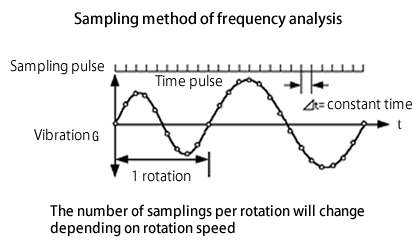Fig. 2

On the other hand, if sampling is performed using a sampling clock synchronized with the rotation speed, for example, a signal of 64 pulses per rotation, the number of signal samples per rotation does not change even if the rotation speed changes (see Fig. 3 below).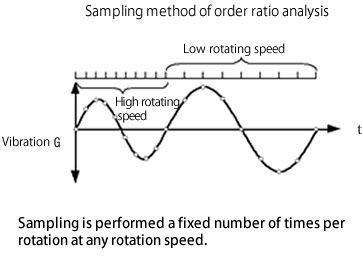Fig. 3

When FFT analysis is performed on the vibration / sound signal sampled in the clock synchronized with the rotation speed, the unit of the X axis is not frequency (Hz) but order (Order). The data displayed as power spectrum of order component is called as rotational order ratio analysis.

1-2 Rotational order ratio and its frequency

The rotational 1st order is a component which completes one cycle for each rotation of the reference body of rotation. The rotational 2nd order is a component which completes two cycles for each rotation. When converting order into frequency, for example, the rotational 1st order for a body of rotation with rotational speed of 600 r/min is 10 Hz, with rotational speed of 900 r/min is 15 Hz as calculated below.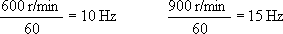The frequency changes with the variation of the rotational speed. However, considering frequency as order, order is a unit that is not affected by the variation of rotation speed. This is the point of rotational order ratio.

1-3 Rotational order ratio analysis vs Frequency analysis when rotation speed changes

The following two figures show color three-dimensional display when the rotation speed is increased and changed. Figure 4 shows order ratio analysis taking the order to the X-axis, while figure 5 shows frequency analysis(The scale of the spectrum is represented by color, and the larger the value becomes, the more blue → yellow → red.)

· Rotational order analysis(color three-dimensional display)

 The same order component is displayed at a constant position of X-axis regardless the change of rotational speed.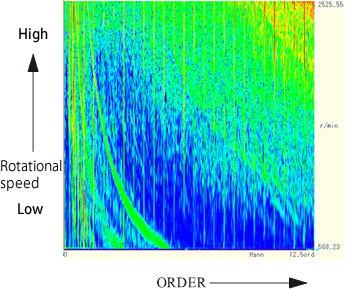Figure 4

· Frequency analysis(color three-dimensional display)

 The frequency of the same order component changes according to rotational speed.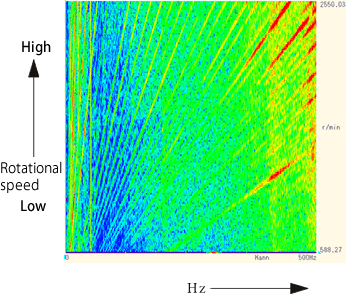Figure 5

The same order component is displayed vertically on X-axis as Fig.4 shown since X-axis represents order. The same order component is displayed in upper right direction as Fig.5 shown since frequency increases proportionally to rotational speed. The yellow/red portion in the above figures shows how they look.

1-4 Sampling signal for order ratio analysis

■External sampling clock and Rotation detector

In the case of normal frequency analysis, sampling clock frequency is 2.56 times of frequency range (maximum frequency to be analyzed). Similarly, in the case of order ratio analysis, the number of sampling clocks per rotation must be 2.56 times the maximum order. The maximum order of our equipment are 6.25/12.5/25/50/100/200/400/800, the number of sampling clock are shown in the following table. Our tracking Analysis Function has a function to automatically divide and multiply the required sampling pulse as below according to the set maximum order analysis by setting the number of output pulse (1 P / R, etc.) per rotation of the rotation detector. This sampling pulse synchronize with rotation as mentioned before.

 Max. order analysis Number of sampling per rotation 6.25 12.5 25 50 100 200 400 800 16 32 64 128 256 512 1024 2048

1-5 Order analysis resolution

The frequency resolution of the frequency analysis by the internal sampling clock is 1/400 of the set frequency range when the analysis data length is 1024 points, it is 1/800, when 2048 points. For example, set 1 kHz as frequency range, frequency resolution is 2.5 Hz= 1000/400 (sampling points 1024). It means you can read spectrum every 2.5 Hz. When order ratio analysis is performed by external sampling clock, the relationship between the maximum analysis order and its resolution is calculated as follow: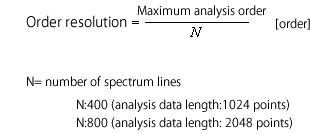(1)

Order resolution can be obtained by the above equation (1) without considering rotation speed. Next, let’s see when order resolution is converted to frequency. For example, if the analysis data length is 1024 points and the maximum analysis order is 100th, order resolution will be 2.5 Hz at 600 r/min and 25 Hz at 6000 r/min in proportion to the rotation speed. When order resolution is considered in order units, it is constant regardless of the rotation speed as in equation (1), but when converted to frequency units, it changes in proportion to the rotation speed as in equation (2) and (3). Order ratio analysis is called as "constant ratio" while, frequency analysis is called as "constant width"

 Conversion from Order resolution to Frequency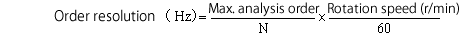• Rotation speed 600 r/min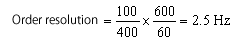(2) • Rotation speed 6000 r/min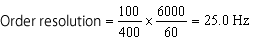(3)

1-6 Aliasing

In the case of order analysis, aliasing may occur as in frequency analysis. Please refer to the remarks below about Aliasing.
Let’s talk about Aliasing. If maximum order is M, rotation speed is N r/min, frequency fx of maximum order is obtained from equation (4). When external sampling mode, sampling frequency fs is automatically set as 2.56 times of frequency fx of maximum order. At that time, anti-aliasing low-pass filter (digital filter) also works in conjunction with sampling frequency, thus Aliasing does not normally occur.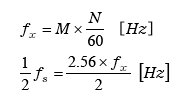(4)

Let’s see the case that anti-aliasing low-pass filter doesn’t link to sampling frequency but fixed.
If the cut-off frequency of low-pass filter is set as 1000 Hz and rotation speed is set as 2400 r/min, frequency fx of 25th order is 1000 Hz as calculated by Equation (5). 1/2 fs is 1280 Hz as same. 1000 Hz or more components are cut by low-pass filter, then Aliasing doesn’t occur.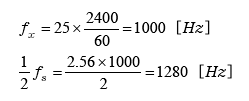(5)

Next, the cut-off frequency of low-pass filter is set as 1000 Hz and rotation speed is set as 1000 r/min, frequency fx of 25th order is 416.7 Hz, 1/2 fs is 533.4 Hz. Components between 533 Hz and 1000 Hz (low-pass filter) are subject to Aliasing. Therefore, if signal components such as vibration noise exist in this frequency band and the cutoff frequency of the low-pass filter is set to 1000 Hz, Aliasing will occur.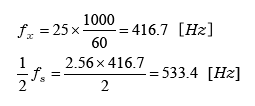(6)

When performing analysis while changing the rotation speed, Tracking Analysis Function is equipped with a tracking low-pass filter whose filter cutoff frequency changes according to the rotation speed.

 What is Aliasing? According to the sampling theorem, it is necessary to sample at a sampling frequency more than twice the highest frequency component fm of the signal. The frequency which is 1/2 times the sampling frequency is referred to as Nyquist frequency. If the original signal contains frequency band fm which is higher than Nyquist frequency,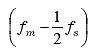component appears at the frequency position folded around fs in the frequency spectrum. It is called as Aliasing. Anti-aliasing filter is equipped for preventing aliasing errors to cut 1/2 fs or more signal.